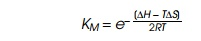Home | | Genetics and Molecular Biology | Entropy, a Basis for Lambda Repressor Inactivation

# Entropy, a Basis for Lambda Repressor Inactivation

The binding of dimeric repressor to operator can be described by a dissociation constant that is related to the change in the free energy via the standard thermodynamic relationship:

Entropy, a Basis for Lambda Repressor Inactivation

The binding of dimeric repressor to operator can be described by a dissociation constant that is related to the change in the free energy via the standard thermodynamic relationship:where KD is the dissociation constant of the dimer from operator, DG is the change in free energy, R is the gas constant, T is the temperature, DS is the change in entropy, andDH is the change in enthalpy.

It is natural to assume that if monomeric repressor were binding to operator, then roughly half as many contacts would be formed between

repressor and DNA and roughly half as many water molecules and ions would be displaced from DNA and the protein as the repressor bound. Therefore we might write for the dissociation constant of the monomer where KM is the dissociation constant for monomer,or KM= KD1/2. This is not correct, however. To make this clear, let DS be written as the sum of the entropy changes involved with the contacts and displacement of water, DSinteraction dimer plus the change in entropy involved with immobilization and orientation of the dimeric repressor,

DSintrinsic dimer:

DSdimer=DSinteraction dimer+DSintrinsic dimer.

The same type of equation can be written for the monomer:

DSmonomer=DSinteraction monomer+DSintrinsic monomer.

Roughly the monomer makes half as many interactions as the dimer and displaces half as many water molecules and ions as it binds. Therefore

DSinteraction monomer=DSinteraction dimer/2.

The same is not true of the intrinsic entropies. The entropy change associated with bringing repressor monomer to rest on operator by correctly positioning and orienting it is nearly the same as the change associated with the dimeric repressor. Thus

DSmonomerâ‰  DSdimer/2,

Figure 14.18 Why a dimer can bind much more tightly than a momomer.Almost all the additional DH provided by the second monomerâ€™s binding can go into increasing DG, whereas for binding of a monomer, almost no DG is left over to contribute to binding.and detailed calculations based on statistical mechanics or experiments show that the inequality can be severe. This is another example of the chelate effect discussed earlier. In other words, a sizable fraction of the total entropy change involved with repressor binding to DNA is associ-ated with its correct positioning. Roughly the same entropy is required to orient a monomer or dimer, but in the case of lambda repressor, the presence of the second subunit of the dimer adds twice as much to the binding energy. Most of this additional energy can go into holding the dimer on the DNA, and hence KD is much less than K2M (Fig. 14.18).

Study Material, Lecturing Notes, Assignment, Reference, Wiki description explanation, brief detail
Genetics and Molecular Biology: Lambda Phage Genes and Regulatory Circuitry : Entropy, a Basis for Lambda Repressor Inactivation |